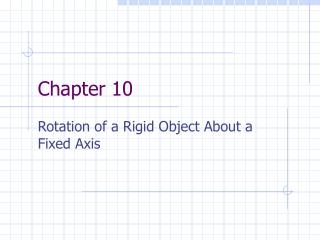DownloadDownload PresentationChapter 10

# Chapter 10

Download Presentation## Chapter 10

- - - - - - - - - - - - - - - - - - - - - - - - - - - E N D - - - - - - - - - - - - - - - - - - - - - - - - - - -
##### Presentation Transcript

1. Chapter 10 Rotation of a Rigid Object About a Fixed Axis

2. Rolling Motion • Rigid body—object or system of particles in which distances between component parts remains constant • Translational motion—movement of the system as a whole • Described by the motion of the center of mass • Rotational motion—movement of individual parts around a particular axis • The spinning

3. Angular Measure • Circular system of position measurement • Useful when objects move in circular (or partially circular) paths • Planetary motion (orbits) • Useful when objects are rotating • Planetary motion (rotation)

4. Angular measure • Rectangular (Cartesian) coordinates • x—perpendicular distance from the vertical axis • y—perpendicular distance from the horizontal axis • Angular (circular) coordinates • If a line is drawn directly from the origin to any point . . . • r—distance from the origin • --angle made with the horizontal • Units of degrees or radians • x = rcos • y = rsin

5. Angular measure • Displacement • x or y = linear displacement •  = angular displacement • For a circle • r = 0, so only angular displacement is needed to describe motion

6. Angular measure • Radians • s = arclength • Distance around a circular path • 1 radian = angle that produces an arclength equal to r • 1 rad = 57.3 • Degrees • 1 revolution = 360 = 2 rad • 1 = 60 minutes • 1 minute = 60 seconds • *parsec

7. Angular measure • Angular speed () • Units of rad/s • rpm—revolutions per minute • Common unit, but not standard metric • Angular velocity () • Direction given by right hand rule • Curl fingers in direction of rotation () • Thumb points in direction of 

8. Angular measure • Instantaneous linear velocity (v) is in a straight line tangent to the circular path • v = r • For constant angular velocity, tangential (linear) velocity increases with the distance from the origin • How CD’s work

9. Angular measure • Period (T)—time required for 1 complete revolution (cycle) • Units of seconds (s) • Frequency (f)—number of revolutions completed every second • Units of 1/s, s-1, cycles/s • Renamed Hertz (Hz)

10. Angular Kinematics • Angular acceleration () = rate of change of angular velocity • Does not apply to Uniform Circular Motion • Related to linear (tangential) acceleration Example 7.10, p. 225

11. Angular Kinematics • Angular Kinematic Equations

12. Rolling motion • Rolling without slipping—surface do not move with respect to the point of contact • Direct relationship between rotational and translational motion • s = distance traveled by the center of mass • vcm = velocity of the center of mass • acm = acceleration of the center of mass

13. Torque • Force is the cause of translational acceleration • Torque() is the cause of rotational acceleration • Units of m*N or N*m (not Joules) • The meter-Newton or Newton-meter • Moment arm (r)—distance from the axis of rotation to the the point where force is applied • Only component of force perpendicular to moment arm contributes to rotation Muscle Torque

14. Equilibrium • Translational equilibrium • F = 0 • No net force • Rotational equilibrium •  = 0 • No net torque • Static Equilibrium—no movement • Kinetic Equilibrium—constant velocity See-saw Meter stick, page 258

15. Rotational Dynamics • Newton’s 2nd Law (translational) • F = mass x acceleration • Newton’s 2nd Law (rotational) •  = rotational equivalent of mass x rotational equivalent of acceleration

16. Rotational Dynamics • I = moment of inertia • Rotational equivalent of mass • Distribution of mass • Dependent on axis of rotation common geometrical shapes, p. 304

17. Moment of Inertia

18. Moment of Inertia

19. Rotational Kinetic Energy • Remember, all your translational equations have rotational equivalents • Rotational work • Rotational KE • Rotational Work-Energy Theorem